﻿ Dynamic Analysis of Manipulator Arm for 6-legged Robot

Dynamic Analysis of Manipulator Arm for 6-legged Robot

Ivan Virgala, František Šimčák, Zdenko BobovskýOPEN ACCESSPEER-REVIEWED

Dynamic Analysis of Manipulator Arm for 6-legged Robot

Ivan Virgala1,, František Šimčák1, Zdenko Bobovský2

1Department of applied mechanics and mechatronics, Technical University of Košice / Faculty of mechanical engineering, Košice, Slovakia

2Department of Robotics, Technical University of Ostrava / Faculty of mechanical engineering, Ostrava, Czech Republic

Abstract

The paper deals with dynamic analysis of manipulator arm which is part of 6-legged robot. The robot is designed for search and rescue purposes like searching for survivors after building collapse or earthquake. The manipulator arm has tentacle as end-effector which serves for grasping and transfer of ruins. The aim of the paper is investigation of particular dynamic reactions in certain nodes of the manipulator links in order to optimization purposes. The results of simulations then can be used for FEM analysis. For dynamic analysis software SolidWorks and Matlab / SimMechanics are used.

At a glance: Figures

1234
Prev Next

• Virgala, Ivan, František Šimčák, and Zdenko Bobovský. "Dynamic Analysis of Manipulator Arm for 6-legged Robot." American Journal of Mechanical Engineering 1.7 (2013): 365-369.
• Virgala, I. , Šimčák, F. , & Bobovský, Z. (2013). Dynamic Analysis of Manipulator Arm for 6-legged Robot. American Journal of Mechanical Engineering, 1(7), 365-369.
• Virgala, Ivan, František Šimčák, and Zdenko Bobovský. "Dynamic Analysis of Manipulator Arm for 6-legged Robot." American Journal of Mechanical Engineering 1, no. 7 (2013): 365-369.

 Import into BibTeX Import into EndNote Import into RefMan Import into RefWorks

1. Introduction

In general, there are two basic approaches for establishing dynamic model of manipulator, namely Newton - Euler and Euler - Lagrange. Newton - Euler approach carry out a detail force and torque analysis of each rigid link with some physical knowledge such as Newton’s third law, and then apply Newton’s law and Euler’s equation to each of the rigid links to obtain a set of 2nd order ODE in the position and angular representation of each rigid link . The dynamic model obtained by Euler - Lagrange approach is simpler and more intuitive, and also more suitable to understand the effects of changes in the mechanical parameters. The links are considered together, and the model is obtained analytically. The disadvantage of Euler - Lagrange method is its computationally inefficiency . It should be note, that this approach starts be very difficult when the number of manipulator links increases. The more links manipulators has, the more complicated the dynamic model is, because of difficulty of differential equations. In these cases the possibility of occurrence of errors arises and therefore it is more effective to use certain software for building of dynamic model. By means of software the user works with block diagrams representing particular joints, bodies, constrains etc. This kind of approach is so-called Model-based design and it is very useful tool especially for difficult configurations of mechanisms.

The aim of the paper is determination of reaction forces in certain nodes of manipulator arm links. Based on this results can be manipulator design optimized by following FEM analysis.

The paper is divided into following sections: at first, the workspace of manipulator arm is investigated based on direct kinematic model consisting of homogeneous transformation matrices. In the third section the trajectory for particular joints is planned. This trajectory for dynamic model is used in section 4. In SolidWorks the 3D model of manipulator arm is designed and parameters like mass and moment of inertia are obtained. These parameters are necessary to building model in Matlab / SimMechanics. The results of reaction forces affecting manipulator arm link in the figures are shown.

2. Workspace of Manipulator Arm

The workspace of a manipulator is the total volume swept out by the end-effector as the manipulator executes all possible motions. The workspace is constrained by the geometry of the manipulator as well as mechanical constraints on the joints. For example, a revolute joint may be limited to less than a full 360° of motion . By determination of workspace user knows if kinematic configuration of manipulator is sufficient for his required tasks and operations with manipulator.

In our study, the kinematic model of manipulator arm on the homogeneous transformation matrices is based.

2.1. Manipulator Arm

CAD model of manipulator arm in the Figure 1 is shown.

3D model of manipulator arm in SolidWorks is done.

The manipulator arm consists of two links, end-effector and four hydraulic motors. A material of manipulator links is steel 11 523. For mathematic model will be the links considered as rigid bodies. In the Figure 2 the kinematic configuration of manipulator is shown. Based on this configuration the direct kinematic model can be derived.

As can be seen from the Figure 1 the rotation motion of manipulator links is ensured by prismatic joints of hydraulic motors. Extension of motors is from 0 to 200 mm. Range of movement of particular joints is:

q1 <0°; 360°>, q2 <5°; 65°>, q3 <38°; 137°> and q4 <2°, 88°>.

2.2. Direct Kinematic Model

By direct kinematic model can be determined the end-effector position by knowing of all general variables q. By considering of particular transformation matrices (1 - 6) we obtain final transformation matrix (7) by product of these matrices. Final transformation matrix (7) determines position and orientation of end-effector in global coordinate system x0, y0, z0.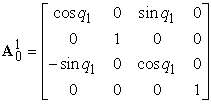(1)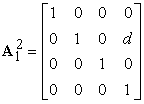(2)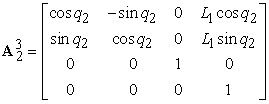(3)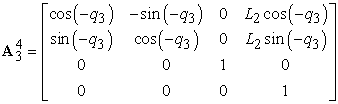(4)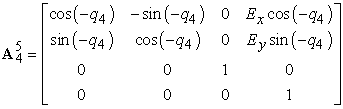(5)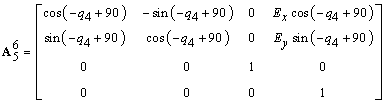(6)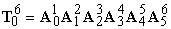(7)

Final transformation matrix (7) is squared matrix with dimension 4x4. The first, second and third element in last column of this matrix represent position of end-effector. By determining of direct kinematic model the workspace of manipulator arm can be plotted. For this purpose the suitable recursive algorithm in Matlab was written. The step of particular joint motion in this algorithm is 10° - 15°. For more precise plotting of workspace the step should decreases.

The workspace of manipulator arm in the Figure 3, Figure 4, Figure 5 is shown.

From the Figure 4, Figure 5 it is obvious that range of motion from the axis of rotation around z to the last point of end-effector, in x-axis direction is roughly 3 m. It should be note that this range is so-called reachable workspace and it is only theoretical. The workspace is often broken down into a reachable workspace and a dexterous workspace. The reachable workspace is the entire set of points reachable by the manipulator, whereas the dexterous workspace consists of those points that the manipulator can reach with an arbitrary orientation of the end-effector. Obviously the dexterous workspace is a subset of the reachable workspace .

3. Trajectory Planning for Manipulator Arm

To achieve required motion of actuators there should be planned the trajectory of motion. For design the trajectory with required position, velocity and acceleration there has to be used polynomial of 5th order. Let´s consider following equation .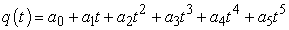(8)

By derivation according time we obtain polynomial for velocity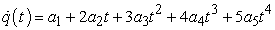(9)

By next derivation we obtain polynomial for acceleration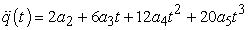(10)

By determination of initial and terminal conditions for position, velocity and acceleration we obtain 6 equations with 6 unknown constants a0, a1, a2, a3, a4, a5. Let´s consider for initial position the zero position, zero velocity and zero acceleration. Initial time is 0 s.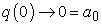(11)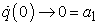(12)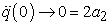(13)

For terminal conditions let´s again consider zero velocity and zero acceleration. Final position is qF.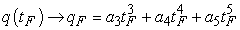(14)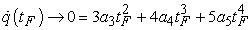(15)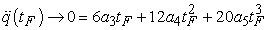(16)

Now, we have 6 equations with 6 unknown constants. Using Matlab the constants a0, …, a5 can be calculated. As final position is considered the maximal extension of hydraulic piston, what is 200 mm. Final time is chosen to be 10 seconds. The solution of equations (11) – (16) is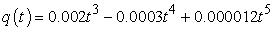(17)

The course of position, velocity and acceleration of hydraulic piston in the Figure 6 is shown.

Trajectory according the Figure 6 is trajectory for one of the hydraulic piston. For the second can be trajectory planned by similar way. It is logical, that the shorter time the piston will moves according planned path, the higher dynamic reactions will arise in this joint. From this reason the prismatic motion of hydraulic piston lasts 10 seconds, see Figure 6. The time of trajectory depends on requirements of performing operation.

Now, planned trajectory of particular hydraulic pistons will be used as input to the mechanic system.

4. Simulation of Manipulator Arm Motion Using Required Trajectory

The simulation manipulator arm motion in Matlab / SimMechanics - First Generation is done. SimMechanics is strong tool for investigation of mechanic or mechatronic systems. It significantly facilitates task of dynamic analysis especially in the case of redundant mechanisms. .

The input to joint actuator is required position, velocity and acceleration of hydraulic piston, which in the section 3 were determined. In this study we do not investigated behavior of manipulator using feedback control. The focus of study is just investigation of dynamic reactions affecting the particular links of manipulator. The task when position, velocity and acceleration are known and the torques have to be determined is task of inverse dynamics. Solution of inverse dynamics is determination of particular torques or forces, which are necessary to reach required position, velocity and acceleration. .

In the Figure 7 the simulation scheme from SimMechanics is shown.

In the study there was investigated motion of manipulator arm lifting the object with mass 200 kg.

The simulation process in the following figures is shown.

In the Figure 8 the sequence of motion can be seen. During the simulation each of manipulator link moves according to different trajectory.

Now, for our study it is important to know the reactions which affect manipulator arm link in the nodes CS1, CS2, CS3 and CS4, see Figure 9. Using block Joint Sensor the reaction in particular axis can be determined.

In the following figures the course of reaction forces in the nodes CS1, CS2, CS3 and CS4 are shown.

Figure 10. Courses of reaction forces in chosen manipulator link nodes

For faster motion of the manipulator link the reaction forces should be higher. By the same way should be done analysis for second manipulator link or any part of manipulator arm. These analyses can be used for optimization analysis in order to redesign manipulator links, if it should be necessary. By means of FEM analysis we can obtain results if the design of links is suitable for required tasks. For FEM analysis the reaction forces will be considered as maximal reaction forces arising during required motion of joints.

5. Conclusion

In the paper manipulator arm of six-legged robot is investigated. The workspace of manipulator can be analyzed by direct kinematic model consisting of homogeneous transformation matrices. For dynamic analysis trajectory of particular joints is planned. For simulating mechanic system Matlab / SimMechanics is used. In our study only reaction forces in contact points of manipulator link are investigated. If reaction forces are too high, the trajectory of particular joints should be lasts longer. The dynamic analysis is done because of using results for design optimization purposes. By FEM can be manipulator links optimized for required operations.

Acknowledgement

The authors would like to thank to ITMS: 26220220141 “Research of modules for intelligent robotic systems” 01/2011-12/2014.

References

  Yiu, Y. K., On the Dynamics of Parallel Manipulators, Proceedings of the 2001 IEEE International Conference on Robotics & Automation Seoul, Korea, May 2001.In article PubMed  Melchiorri, C., Dynamic model of manipulator, Industrial Robotics (Robotics and Automation), University of Bologna.In article  Spong, M. W., Hutchinson, S., Vidyasagar, M., Robot Modeling and Control, John Wiley & Sons, INC 2005.In article  Biagiotti, L., Melchiorri, C., Trajectory planning for Automatic Machines and Robots, Springer 2008.In article  Kordjazi, H., Akbarzadeh, A., Inverse dynamics of a 3-prismatic-revolute-revolure planar parallel manipulator using natural orthogonal complement, Journal of Systems and Control Engineering, Vol. 225, 2010.In article